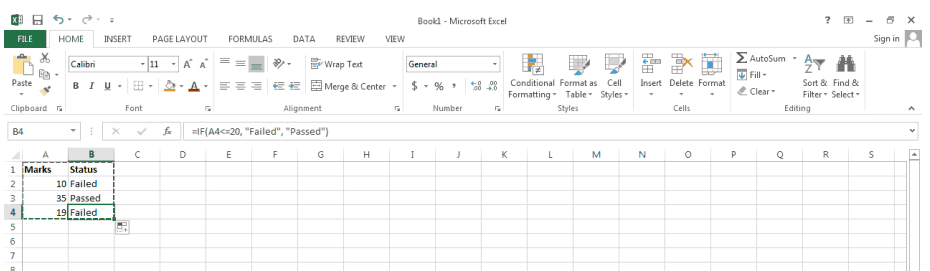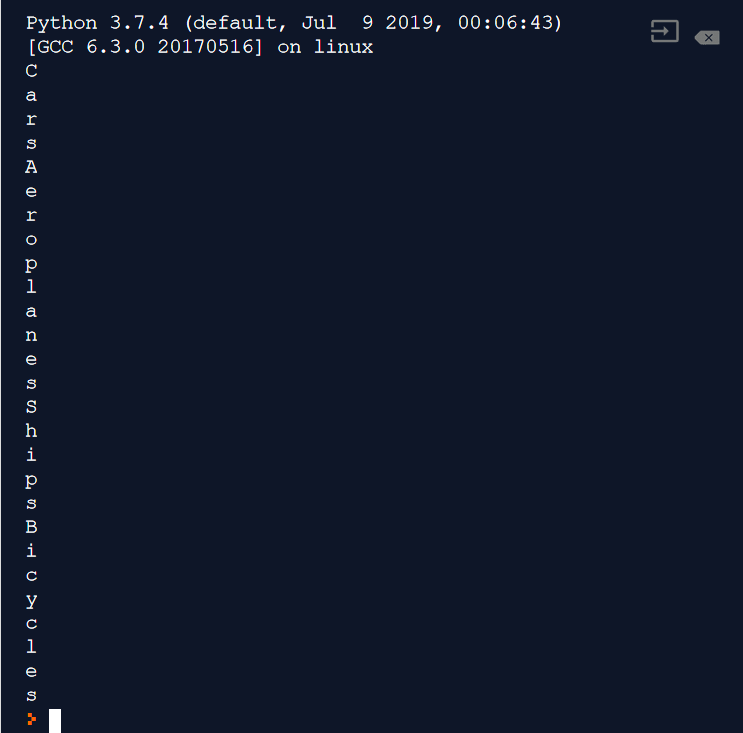Microsoft Excel is a spreadsheet software that’s a part of the iconic Microsoft Office software. With Microsoft Excel, you can store and work with a large database. Excel provides you with various tools that you can use to handle data and sort it. You can use functions, graphical charts, tables and some data analysis tools to make your data presentable and understandable.

Microsoft Excel provides comparison operators that can be combined with functions, which will allow you to automate certain data calculations. Some of these operators include the equals operator (=), the less than operator (<), the greater than operator (>), the less than or equal to operator (<=), the greater than or equal to operator (>=) and finally the not equal to (<>) operator.

We’re going to be using MS Excel 2013 for our tutorial. The differences between Excel 2007, 2010 and 2013 are cosmetic and so you can use any one of the three you have access to. We’re assuming that you’re at least slightly familiar with MS Excel. Don’t worry if you’re not. Excel is pretty intuitive and a basic course in Excel should be enough to get you started.

## The Not Equal to <> Comparison Operator

In this tutorial, you’ll get to learn about the Not Equal to Comparison operator. The symbol for the not equal to comparison operator is “<>”. If you pair it with the IF logical function, you can create all kinds of complex queries. But before we get to the not equal to comparison operator, we’ll take a quick look at the syntax of the IF function. This will help you grasp the concept of the “not equal to” comparison operator better.

The IF Logical Function

The IF logical function is very useful. You will see it used a lot in a typical work environment. For example: you can create an Excel spreadsheet for your school to help you see how the students fared this semester. If a student gets less than 20 out of 50, he fails. If a student gets more than 20, he passes. The syntax for the IF function is:

`IF (logical_test [value_if_true], [value_if_false])`

Explanation: The “logical_test” is where you provide the condition using the comparison operators. For example, did the student score less than 20 marks?  The “value_if_true” part lets you decide what happens if your condition is passed. You can ask excel to display “Student has passed” if the condition is met. The “value_if_false” lets you decide what happens if your condition is failed. You can ask excel to display “Student has failed” if the value of the “logical_test” turns out to be false.

Example: We name our column “A” as Marks and column “B” as Status. We apply the formula =IF(A4<=20, “Failed”, “Passed”) for the entire column B. Now, when we input the marks of the student in column A, we directly get the result in column B.```Marks Status
10 Failed
35 Passed
19 Failed```

Let’s see what happened here. Excel first checked if score of the student was less than or equal to (<=) 20. If it was less than 20, it printed “Failed” in the corresponding cell. If it was more than 20, it printed “Passed”.

Quick Tip: Don’t waste time typing formula for each cell in your column! If you type a formula for a single cell, you can just click on it to select it and drag it downwards by clicking on its outline (A little “+” symbol will appear). This will apply the formula for multiple cells in your column.

## Pairing the Not Equal to Comparison Operator with the “IF” logical function

The not equal to comparison operator is slightly tricky to use. We’ll show you a few ways in which you can use the operator with the IF function. The not equal to operator uses the greater than and less than signs together “<>” together. The general syntax of the not equal to operator is:

`=IF (cellname <> condition, result 1, result 2)`

Let’s continue with our earlier example. Suppose that you want students who have received 20 out of 50 on their exams to be put on probation. You can assign that status using the not equal to comparison operator.

```Marks Status Probation
10    Failed No
35    Passed No
19    Failed No
20    Passed Yes```

We add a third column called “Probation”, and paste this formula into all rows =IF(A#<>20, “No”, “Yes”)  As you can see, the formula is a little tricky. If the value of A is not equal to 20, then the student is not on probation. If the value of A is equal to 20, he is on probation. We’ve also changed our formula for the “Status” column a little. The new formula is =IF(A#<=19, “Failed”, “Passed”). This slight alteration in our formula allows us to give a “Passed” status to any student who has scored 20 on their exams.Quick Tip: How do you use multiple “IF” functions together? You will, in reality, be using several “IF” functions chained together in a real spreadsheet. You can write an “IF” Function within another (or several “IF” functions). For example, if you wanted the students who scored more than 40 to receive the “A” grade, students who scored more than 30 the “B” grade and students who scored more than 20 the “C” grade, students who scored “20” the D grade and students who got less than 20 the “F” grade, you would use the formula =IF(A1>=40, “A”, IF(A1>=30, “B”, IF(A1<>20, “D”,”F”)))

```Marks Status Probation Grade
10    Failed No        A
35    Passed No        F
19    Failed No        B
20    Passed Yes       D```Note that we have not used the “not equal to” comparison operator in assigning grades, because it’s much simpler and faster to use the other operators provided by Excel. There are usually multiple ways you can filter your data. You can choose which method best suits your needs.

As you can see, it has been pretty easy to use the “not equal to” comparison in Excel. We hope these examples give you a good feel of how to use the “not equal to” comparison, and also how to build complex “IF” conditionals. If you’d like to explore Excel further, feel free to take up a Basic Excel course, or even a deep dive with an advanced course.

Page Last Updated: December 2013